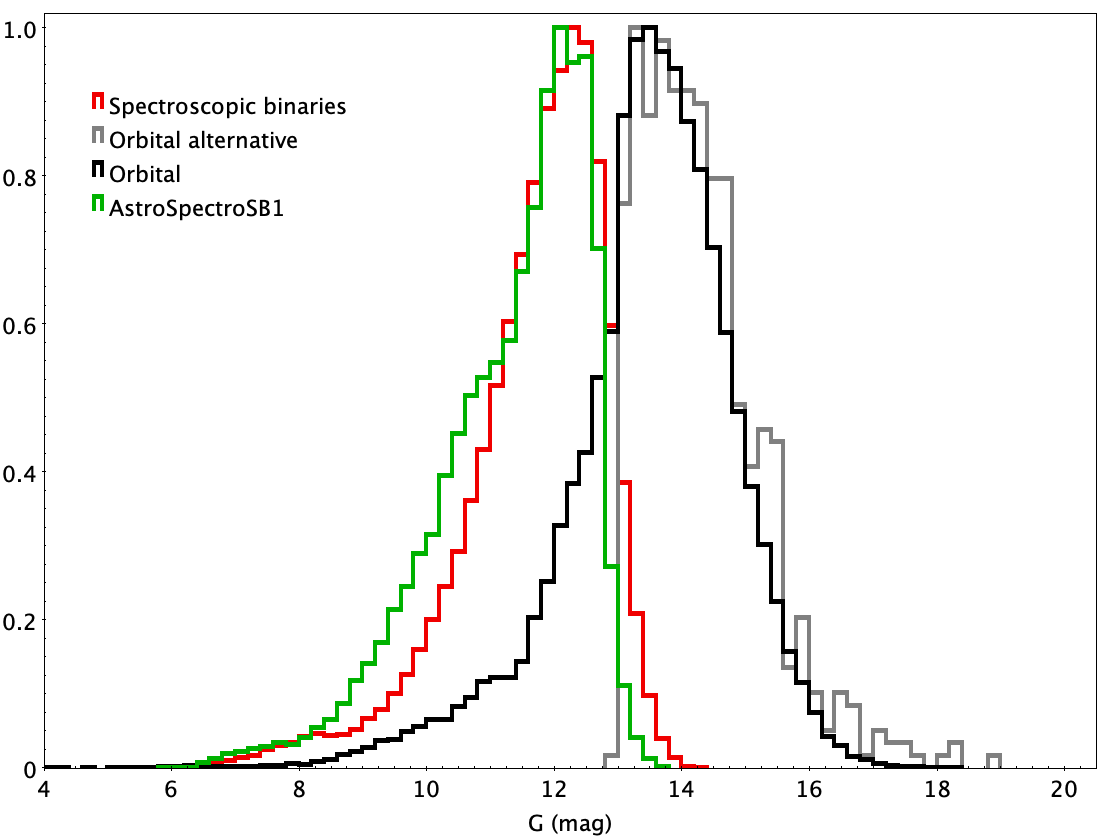# 7.7.1 Introduction

A fraction of sources studied by the upstream pipelines have been identified to fit in more than one orbital models. The aim of the combined solutions pipeline is to find the solutions that are common and to produce an updated solution in order to improve the precision. In practice, however, the three channels astrometric, spectroscopic, eclipsing may find solutions on a limited range of period only, as the order of the three channels is mostly the one by decreasing period. Indeed, chances are very low that a pair with an astrometric orbital solution, i.e. with a large separation may also be eclipsing. Consequently one may either have to combine pairs with small spectroscopic periods which happen to also be eclipsing, or, more frequently, pairs with a large spectroscopic period also having an astrometric orbital solution. In this data release only these two types of combinations have then been treated and are named respectively EclipsingSpectro and AstroSpectroSB1.

Due to the specifications of the radial velocity spectrometer the spectroscopic binaries have a magnitude range brighter than the one of astrometric and photometric binaries. Figure 7.57 shows the normalised magnitude distributions between the different combination models. The small overlapping magnitude range explains the relative small number of combinations.Figure 7.57: (a): Normalised magnitude distribution of spectroscopic binaries (all models), astrometric binaries with orbital model (Orbital and OrbitalAlternative) and combined AstroSpectroSB1 binaries. (b): Normalised magnitude distribution for SB1 spectroscopic binaries, eclipsing binaries and combined EclipsingSpectro.